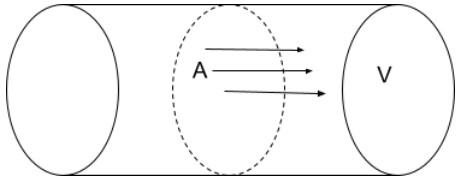# Flow Rate Calculator

Enter the values of Velocity and Area in the respected boxes to find the pipe flow rate of a fluid by using flow rate calculator.

m2
m/s

Give Us Feedback

Flow Rate Calculator helps to calculate the flow rates of water and other fluids passing through a cross-sectional area. It uses the velocity of the fluid and the area of the place it passes through.

This pipe flow calculator can calculate:

• Flow Rate
• Flow Velocity
• Flow Area

## What is Flow Rate?

Flow rate is defined as

The volume of fluid each second that is passing through a cross-sectional slice of the pipe.## Flow Rate formula

The flow rate formula is

Q = vA

Where,

A = cross-sectional area of the pipe,

v = velocity

## Finding the flow rate of fluid

Let’s find out how to calculate flow rate manually.

Example:

Find the flow rate of a fluid through an area of 0.7 m2 flowing with a velocity of 3 m/s.

Solution:

Step 1: Write down the values.

A = 0.7 m2

V = 3 m/s

Step 2: Place the values in the flow rate formula

Q = Av

= 0.7 × 3

Q = 2.1 m3/s

### Math Tools